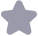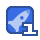### 初学者Python编程实例58例(基础语法很经典)收藏 13

3 f in range(1, 21,2 4 print 'd odg(ab) 0+=a 【程序12】 题日:判断101-200之闫有多少个素数,并输出所有素数 自 1 t!/usr/bin/ python 2+-*-codi:g:utf-8-*- 3 from math import sart 4 def ca-n( for i in range(101, 201) flag k int(sqrt(i)) tor j in range(2, k+l) ifi号j==0 flag 0 11 break f flag == 1 13 print 8d 's(i) 14 15 1f name = main 16 【程序13】 题目:打印出所有的“水仙花数”,所谓“水仙花数”是指一个三位数,其各位数字立方和等于该数本身。例如:153 是一个“水仙花数”,因为153=1的三次方+5的三次方+3的三次方 1 !/usr/bin/python 2 +-*- coding: utf-8 -*- 3 def ran(: for i in range(100,1000) /100 c=(int(i/10))81 ifi==a*+3+bx大3+Cx3: print 05d"o(1) 111f name 12 【程序14】 题目:将一个正整数分解质因数。例如:输入90,打印出90=2*3*3*5 1 +!/usr/bin/python 4 def Ia-n(): n= int(raw input('Enter a number: ) r-nt n h1c(n!=1) for i in range(,n+1) if(ni)==0: n f( print do(i) 13 e⊥se: print 5d * 8 8⊥ f name main 【稈序15】 题目:利用条件运算符的嵌套来完成此题:学习成绩>=90分的同学用A表示,60-89分之问的用B表示,60分以下 用C表示 1 #!/usr/bi 2 3 def ma=n(): ber: ' ) 1fs>=60 B se. 11 nt grade 13 if name 14 .a-n() 【程序17】 题目:输入一行字符,分别统计出其中英文字母、空格、数字和其它字符的个数 1 +!/usr/bin/python 2#一- coding:utf-8-木 3 there is no + operator in Python 4 import string o def ra-n(: s= raw input(input a string: ' lctt≈l space digit =0 e 0 for c in s: ifc.isa⊥pha() 13 elif c isspace(): space+=1 clif c isdigit() didit+= else other+=l print There are od letters, od spaces, od digits and od other characters in your string (letter, space, digit, other 22 if name 1n ra-n() 【程序18】 题目:求s=a+aa+aa+aaa+aaa的值,其中a是个数字。例如2+22+222+222.+2222(此时共有5个数相 加),几个数相加有键盘控制 1 #!/usr/bin /python 2 #-*- codi:Ig: utf-8 -* 3 def ma-n(: basis int(raw input("Input the ba n= int (raw input("Input thc longest lcngth o= numbcr b= basis um =0 783 for i in range(0, n) if i==n-1 print d 5(basis), else print d +8(Casis), 13 sum+=basis 14 basis basis*10+2 t 111 18i士name maIn 19 【程序19】 题目:一个数如果恰好等」它的因子之和,这个数就称为“完数”。例如6=1+2+3编程 找出1000以内的所有完数 1 from math import sart 2 n int(raw input('input a number: ' ) 3 sum = n*-1 4 k =int(sort(n) 5 for i in range(l, k+1): sum += n 9 if sum pr=nt YES I 11 else: print ' NO r 【程序20】 3题日:一球从100米高度自由落下,每次落地后反跳回原高度的一半;再落下,求它在 4 第10次落地时,共经过多少米?第1~次反弹多高 s=100 7h=50.0 8 or i in range(2, 11): h/=2 11 print "the sum length ot path: of s 12 print the last height is: 6=h 11 1 2【程序21】 3题日:猴子吃桃问题:猴子第一天摘下若干个桃子,当即吃了一半,还不瘾,又多吃了一个 第二天早上又将剩下的桃子吃担一半,又多吃了一个。以后每天早上吃了前一天剩下 的一半零一个。到算10天早上想再吃时,见只剩下一个桃子了。求第一天共摘了多少 7n=1 8 tor n range(9,0,-1 (1+1)<<1 10 print n 2★ 3【程序22】 4题目:两个乒乓球队进行比赛,各出三人。甲队为三,b,C三人,乙队为x,y,z三人。已抽签决定 比赛名单。有人向队员打听比赛的名单。a说他不和κ比,c说他不和x,z比,请编稈序找出 三队赛手的名单 8 for i in range(ord('x),ord('z')+ 1): for j in rangc(ord(x),ord('2)+ 1): 10 if 11 for k in range(ord('x), ord(2)+1): i f( k) and k) if(i!= ord(x'))and (k ! ord('x))and (k ord (2)): print r order is a os\t b--%s\tc--os(chr(i), chr(i), chr(k) 2【程序23】 3题目:打印出如卜图案(菱形) 4 7★★★★ 8大大大大大x 大大大 12 13 tor i -n range(1,8, 2) 14 t lo for i -n range(5,0,-2): r=nt 1 2【程序24】 3题目:有一分数序列:2/1,3/2,5/3,8/5,13/8,21/13..求出这个数列的前20项之和。 5u=2,0 d=1.0 8 for i -n range(0, 20) 12 print 'ofs 2【程序25】 3题目:求1+21+3!+.+20!的和 E:t=1 7 for i -n range(1 21) 10 print s 2【程序26】 3题目:利用递归方法求5!。 4 J def fun(i) 1 return 1 return i fun(1-1) 10 prin- fun(5) 2【程序27】 3题目:利用递归函数调用方式,将所输入的5个字符,以相反顺序打印出来。 o def cutput(s, 1): return print s[1-1] itput(s,1-1) 11 s- raw input( Input a string: 121=1en(s) 13 outout(s, 1) 2【程序28】 3题目:有5个人坐在一起,问第五个人多少岁?他说比第4个人大2岁。问第4个人岁数,他说比第 个人大2岁。问第三个人,又说比第2人大两岁。问第2个人,说比第一个人大两岁。最后 问第一个人,他说是10岁。请问第五个人多大? 7 def fun(i) return 10 return fun(i-1)+2 12 print tun(5) 2【程序29】 3题目:给一个不多于5位的正整数,要求:一、求它是几位数,二、逆序打印出各位数字。 3 def fun(i, cnt) 6783 print There are d digit in the number. cnt return print 1510 10 cnt+ fun 13 14 i=int(raw input( Input. a number: ') 【稈序30】 3题目:一个5位数,判断它是不是回文数。即12321是回文数,个位与万位相同,十位与千位相同。 ci -int(raw input('Input a numbcr(10000 99999): ' ) 7ifi<10000ori>99999: print 'Input Error! 9e1 i str(i) flag =0 g in range(0, 2) fi[j]!=i[4 14 flag =1 rca 16 lg 2【程序36】【筛选法】 3题日:求100之内的素数 5a=[0.*101 s土 or l-n range(2,11): for j in range(i+i, 101,1): e for i in range(2, 101) 10 i=a[i]!=-1 11 print 2【稈序37】 3题目:对10个数进行排序 J print ' input 1C numbers s please 61=「1 7 for i in range(10) l append(int(raw input( - nput a numbcr: )) 9#可以直接使用scrt函数:1.sor-() 10#也可以自己写排序代码(选择排序) 11 for i -n range(9 12 for j in range(i+1, 10):

...展开详情XHSoros 不错的教程，适合初学者，正在看，不知道能不能做出点什么来
2019-03-22

2018-12-24

why1673